## Compass Geometry

With compasses alone, all the points that can be constructed with straightedges and compasses can be constructed. That means that straightedges are only necessary for the actual drawing of lines. One would not want to dispense with straightedges, however, since the constructions with compasses alone are much more complicated.

The geometry of compasses was developed independently by G. Mohr in Denmark in 1672, and by L. Mascheroni in Italy in 1797. The easiest way, however, to show that compasses are sufficient depends on circle inversion which wasn't invented until 1828 by Jacob Steiner.

References: R. Courant and H.E. Robbins, What is Mathematics? Oxford Univ. Pr., New York, 1953.
H.S.M. Coxeter, Introduction to Geometry, Wiley, New York, 1961.
D. Pedoe, Circles, Dover, New York, 1957.

There are three methods to construct points in Euclidean geometry.

CC: construct the intersections of two circles given their centers and a point on each circumference
LC: construct the intersections of a line given by two points and a circle given its center and a point on its circumference
LL: construct the intersection of two lines, each given by two points

It is evident that the first construction is possible by compasses alone; just draw the two circles. The other two constructions will be possible if we can show that we can invert points in circles with compasses alone, and find centers of circles. Those are the constructions

PC: constuct the inverse P' of a point P in a circle given its center and point on its circumference
C: construct the center of a circle given three points on its circumference
For then we can just choose an arbitrary circle outside the figure, apply PC to invert in that circle the points in the figure (so that all the circles and lines of data in LC and LL become circles), apply CC to find the intersections, and apply PC to find the corresponding intersections for the original figure. Along the way, we'll have to use construction C to find the centers of the inverted circles since centers of circles aren't preserved under inversion.

#### Construction PC to invert a point in a circle

When A is any geometric figure (point, line, circle, etc.) and C is a circle, we'll use the notation A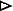C for the result of inverting A in the circle C.

Let there be given a circle C with center O and point A on its circumference, and a point P either outside the circle C or inside but more than half the radius away from O. Here is a construction to invert P in the circle to get P' = PC. Draw the circle with center P and radius PO. Then this new circle meets the circle C at two points, Q and Q'. Draw the two circles with centers Q and Q' and radii QO and QO', respectively. Besides O, they will meet at another point P'.

Then the two triangles QOP and P'OQ are each isosceles, and they have the same base angles, so they're similar. Therefore OP:OQ = OQ:OP'. Thus, P' is the inverse of P in the original circle C.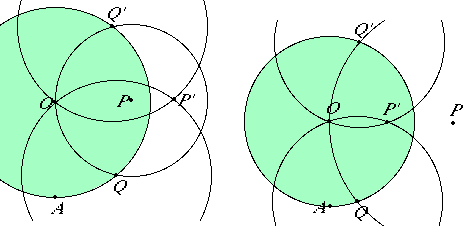Now, this construction doesn't work if P is within half the radius of O since the two circles won't intersect to produce the points Q and Q', so some other construction is needed to invert such P. If P is at least a quarter of the radius away from O, then we can easily find the point R twice as far from O as P is, then invert R in the circle C to get R' = RC by the method described above, and then P' will be the point twice as far from O as R' is. The doubling construction

2OP: given two points O and P, constuct the point R on the line OP twice as far from O as P is
can be effected as follows. Draw the circles with centers O and P and radius OP. Let them intersect at Q and Q'. Draw the circle with center Q and radius QQ'. It will intersect the circle with center P at the points Q' and R.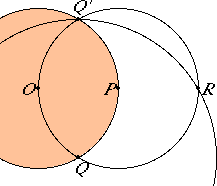Now we can invert a point P in a circle at least a quarter of a radius away from the center.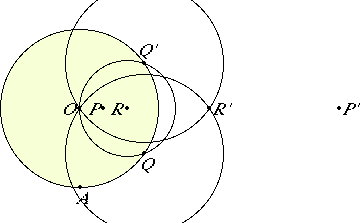When P is very close to O, all we have to do is repeatedly double the distance from O until we've got a point at least half the radius from O, invert that point, and double the resulting distance the same number of times to get P'.

#### Bisecting and multisecting lines

Before going on, we can use inversion in circles to effect a construction to bisect a line segment, or more generally, cut off one n-th of a line segment. To bisect a line segment OP, use construction 2OP to double it to OR, then construction PC to invert R in the circle with center O and radius OP to get the midpoint M of OP.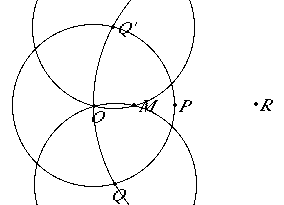We can trisect OP by doubling OP to OR then doubling PR to PS, so that OS is triple OP, then inverting S in the circle with center O and radius OP to get S'. Then OS' will be one-third of OP

More generally, we can cut off an n-th of OP by first extending OP by a factor of n, then inverting in that same circle.

#### Construction C to find the center of a circle given three points on its circumference

We're not done yet in showing that compasses alone are sufficient to construct all the constructable points in Euclidean geometry. The problem is that all the circle constructions depend on knowing the centers of the circles, and when we invert one circle C in another D to get a circle E = CD, then the center of E is not the inverse of the center of C inverted in D. The center of E is determined, however, by three of the points on E, which can by found by taking any three points on C and inverting them in D. Thus, we'll need the construction C to find the center of a circle given three points on its circumference.

Let P, Q, and R be three given points. We are to construct the center O of the circle passing through them. We'll find O by inverting everything in the circle C with center P and radius PQ.

First, invert the point R in C to get the point R' = RC. Next draw two circles, one with center Q and radius QP, the other with center R' and radius R'P. Besides at P, these two circles will intersect at another point O'. This point O' is the reflection of P in the line QR', that is, O' = PQR'. Finally, invert O' in C to get O.

Now when the desired circle PQR is inverted in the circle C, then the result is the straight line QR', since P will be sent to the point at infinity,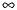. Algebraically, PQRC = QR'. We can now show that O is the center of the circle PQR, that is, O =PQR.PQR = (PC)(QR'C) = (PQR')C = O'C = O.

Thus, we have constructed O, the center of the circle passing through the three points P, Q, and R.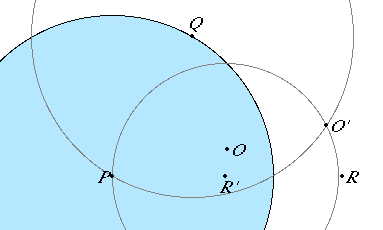April, 1998

David E. Joyce
Department of Mathematics and Computer Science
Clark University
Worcester, MA 01610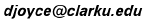My Homepage